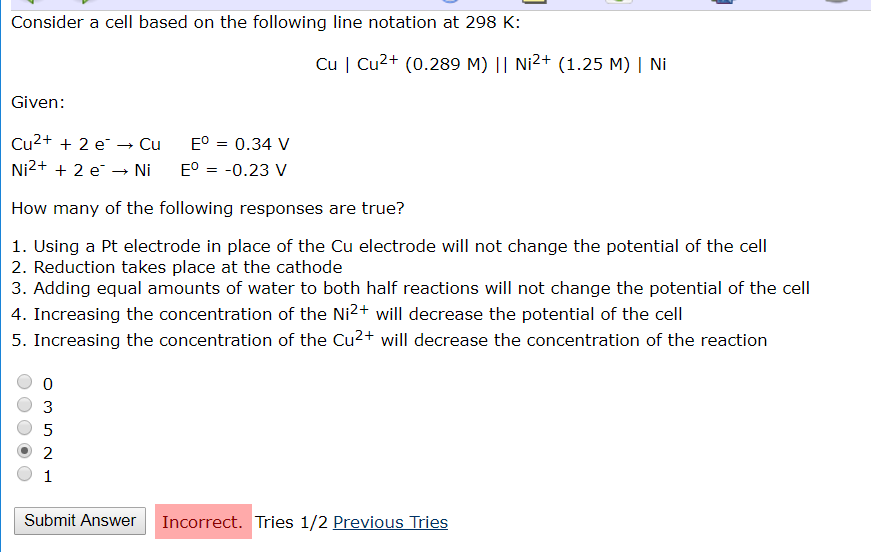# Question & Answer: Consider a cell based on the following line notation at 298 K: Cu | Cu^2+ (0.289 M) || Ni^2+…..Consider a cell based on the following line notation at 298 K: Cu | Cu^2+ (0.289 M) || Ni^2+ (1.25 M) | Ni Given: Cu^2+ + 2 e^- rightarrow Cu E^0 = 0.34 V Ni^2+ + 2 e^- rightarrow Ni E^0 = -0.23 V How many of the following responses are true? 1. Using a Pt electrode in place of the Cu electrode will not change the potential of the cell 2. Reduction takes place at the cathode 3. Adding equal amounts of water to both half reactions will not change the potential of the cell 4. Increasing the concentration of the Ni^2+ will decrease the potential of the cell 5. Increasing the concentration of the Cu^2+ will decrease the concentration of the reaction 0 3 5 2 1